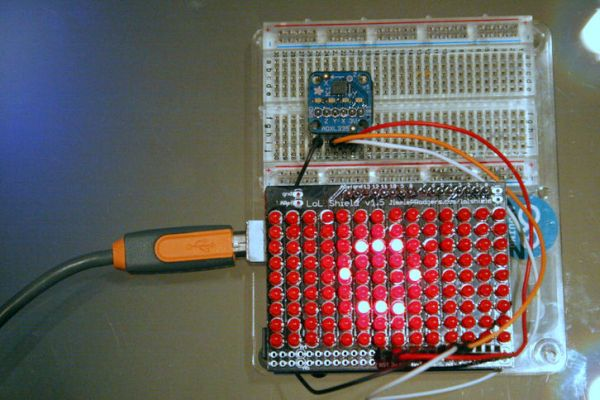# Arduino makes 2D Level

This is a project for Arduino to make a 2D Level, aimed at beginners.

Arduino draws a circle on an LED Matrix that moves around according to readings from a 2D Accelerometer.

Objectives:
* Learn how to draw a circle using simple Maths
* Learn how to use the LOL shield for the Arduino
* Learn how an accelerometer works## Step 1: What you need

You will need:
* Arduino UNO
* LOL shield   (http://jimmieprodgers.com/kits/lolshield/)
* Breadboard for wiring up e.g. (http://www.oomlout.co.uk/prototyping-bundle-for-arduino-ardp-p-186.html)
* Some Wires

## Step 2: Wire it up

Attach the LOL shield to the Arduino.

Wire the Accelerometer as follows:
+3V
GND
X => A0
Y => A1
Z => N/C  (Not Connected)

Note: The LOL shield does not come with Headers (as shown in the picture), attached to the topside.

I bought some here: http://www.amazon.co.uk/gp/product/B004RASBVY/ref=oh_details_o06_s00_i00

## Step 3: Install the LOL Shield Libraries

Install the Arduino libraries for the LOL shield if you don’t have them already.You can get the link to them from this page:
http://jimmieprodgers.com/kits/lolshield/

We’ll start by drawing a circle.This circle will eventually move around as we tilt the device. But first things first….

We’ll illuminate individual pixels on the LOL shield using the LOL library command:

LedSign::Set(x, y , 1);

where x and y are the coordinates on the shield of the LED we wish to illuminate

Our job is to figure out how to calculate the x,y  values that make a circle.

In Pseudo code we do it like this to draw just one quarter (Quadrant) of the circle:

for x = 0 to RADIUS
y=f(x);     // This means y is some function of x. We haven’t said what function yet
plot(x,y)
end

So what’s the function?

r^2 = x^2 + y^2

where x^2 means “x squared” or “x to the power of 2”

We know x because it’s the for loop iterator;
We know r because it’s the radius,
So we re-arrange to find y

y = sqrt(r^2 – x^2)    // sqrt() means square-root

When you code it up you get a circle as shown in the picture.

### Major Components in Project

Arduino UNO
* LOL shield
* Accelerometer

For more detail: Arduino makes 2D Level

#### This Post / Project can also be found using search terms:

• https://duino4projects com/arduino-makes-2d-level/